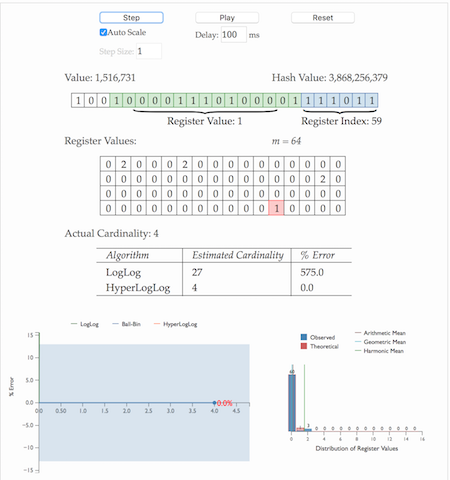# 神奇的HyperLogLog算法

## 基数计数基本概念

1. 当统计的数据量变大时，相应的存储内存也会线性增长
2. 当集合$S_u$变大，判断其是否包含新加入元素$x_i$的成本变大

## 基数计数方法

### B树

B树最大的优势是插入和查找效率很高，如果用B树存储要统计的数据，可以快速判断新来的数据是否已经存在，并快速将元素插入B树。要计算基数值，只需要计算B树的节点个数。 将B树结构维护到内存中，可以快速统计和计算，但依然存在问题，B树结构只是加快了查找和插入效率，并没有节省存储内存。例如要同时统计几万个链接的UV，每个链接的访问量都很大，如果把这些数据都维护到内存中，实在是够呛。

### bitmap

bitmap可以理解为通过一个bit数组来存储特定数据的一种数据结构，每一个bit位都能独立包含信息，bit是数据的最小存储单位，因此能大量节省空间，也可以将整个bit数据一次性load到内存计算。 如果定义一个很大的bit数组，基数统计中每一个元素对应到bit数组的其中一位，例如bit数组 $001101001$代表实际数组$[2,3,5,8]$。新加入一个元素，只需要将已有的bit数组和新加入的数字做按位或 $(or)$计算。bitmap中1的数量就是集合的基数值。

bitmap有一个很明显的优势是可以轻松合并多个统计结果，只需要对多个结果求异或就可以。也可以大大减少存储内存，可以做个简单的计算，如果要统计1亿个数据的基数值，大约需要内存： 100000000/8/1024/1024 $\approx$ 12M

32*100000000/8/1024/1024 $\approx$ 381M

bitmap对于内存的节约量是显而易见的，但还是不够。统计一个对象的基数值需要12M，如果统计10000个对象，就需要将近120G了，同样不能广泛用于大数据场景。

### 概率算法

• Linear Counting(LC)：早期的基数估计算法，LC在空间复杂度方面并不算优秀，实际上LC的空间复杂度与上文中简单bitmap方法是一样的（但是有个常数项级别的降低），都是$O(N_{max})$
• LogLog Counting(LLC)：LogLog Counting相比于LC更加节省内存，空间复杂度只有$O(log_2(log_2(N_{max})))$
• HyperLogLog Counting(HLL)：HyperLogLog Counting是基于LLC的优化和改进，在同样空间复杂度情况下，能够比LLC的基数估计误差更小。

#### HyperLogLog的惊人表现1. 通过hash函数计算输入值对应的比特串
2. 比特串的低 $t(t=log_2^m)$位对应的数字用来找到数组$S$中对应的位置 $i$
3. $t+1$位开始找到第一个1出现的位置 $k$，将 $k$ 记入数组$S_i$位置
4. 基于数组$S$记录的所有数据的统计值，计算整体的基数值，计算公式可以简单表示为：$\hat{n}=f(S)$

1. 为什么要记录第一个1出现的位置？
2. 为什么要有分桶数组 $S$
3. 通过分桶数组 $S$ 计算基数的公式是什么？

## hyperloglog原理理解

1. $n$次伯努利过程的投掷次数都不大于$k_{max}$
2. $n$次伯努利过程，至少有一次投掷次数等于$k_{max}$

$P_n(X \le k_{max})=(1-1/2^{k_{max}})^n$

$P_n(X \ge k_{max})=1-(1-1/2^{k_{max}-1})^n$

$n\ll 2^{k_{max}}$时，$P_n(X \ge k_{max})\approx0$，即当$n$远小于$2^{k_{max}}$时，上述第一条结论不成立；
$n\gg 2^{k_{max}}$时，$P_n(X \le k_{max})\approx0$，即当$n$远大于$2^{k_{max}}$时，上述第二条结论不成立。 因此，我们似乎就可以用$2^{k_{max}}$的值来估计$n$的大小。

## hyperloglog算法讲解

### 分桶平均

HLL的基本思想是利用集合中数字的比特串第一个1出现位置的最大值来预估整体基数，但是这种预估方法存在较大误差，为了改善误差情况，HLL中引入分桶平均的概念。

$5bit*64=32bit$

### 偏差修正

m = 2^b   # with b in [4...16]

if m == 16:
alpha = 0.673
elif m == 32:
alpha = 0.697
elif m == 64:
alpha = 0.709
else:
alpha = 0.7213/(1 + 1.079/m)

registers = *m   # initialize m registers to 0

###########################################################################
# Construct the HLL structure
for h in hashed(data):
register_index = 1 + get_register_index( h,b ) # binary address of the rightmost b bits
run_length = run_of_zeros( h,b ) # length of the run of zeroes starting at bit b+1
registers[ register_index ] = max( registers[ register_index ], run_length )

##########################################################################
# Determine the cardinality
DV_est = alpha * m^2 * 1/sum( 2^ -register )  # the DV estimate

if DV_est < 5/2 * m: # small range correction
V = count_of_zero_registers( registers ) # the number of registers equal to zero
if V == 0:  # if none of the registers are empty, use the HLL estimate
DV = DV_est
else:
DV = m * log(m/V)  # i.e. balls and bins correction

if DV_est <= ( 1/30 * 2^32 ):  # intermediate range, no correction
DV = DV_est
if DV_est > ( 1/30 * 2^32 ):  # large range correction
DV = -2^32 * log( 1 - DV_est/2^32)


## redis中hyperloglog实现

redis正是基于以上的HLL算法实现的HyperLogLog结构，用于统计一组数据集合中不重复的数据个数。 redis中统计数组大小设置为$m=16384$，hash函数生成64位bit数组，其中 $log_2{16834}=14$ 位用来找到统计数组的位置，剩下50位用来记录第一个1出现的位置，最大位置为50，需要$log_2{50}=6$ 位记录。